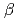Theoretical calculation ofdecay for delayed neutron is studied.decay is one of the nuclear decay processes, but only occurs with the weak interaction. The number of averaged delayed neutron emission is estimated from the idea of the summation method, in which the averaged delayed neutron emission is sum of the-delayed neutron emission probabilities multiplied by cumulative fission yield. Under the consideration of the gross they of thedecay, current status for reproduction of-decay rate and delayed neutron probability is studied.